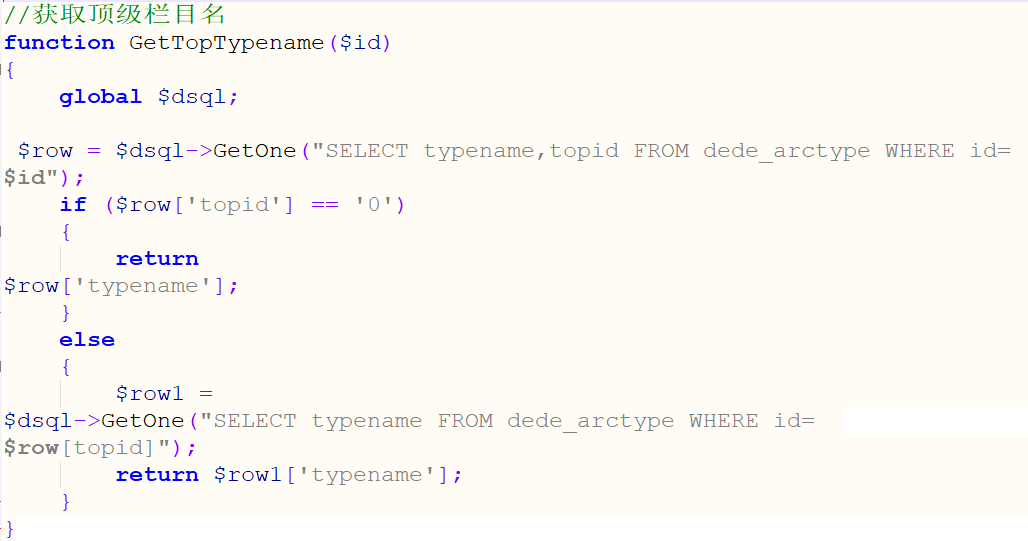织梦dedecms网站更新空白没反应的处理方法2021年2月28日13:19:32 评论 477

{dede:field name='typeid' function="GetTopTypename(@me)"/}

{dede:type}[field:typename/]{/dede:type}

//获取顶级栏目名
function GetTopTypename(\$id)
{
global \$dsql;

\$row = \$dsql->GetOne("SELECT typename,topid FROM dede_arctype WHERE id=
\$id");
if (\$row['topid'] == '0')
{
return
\$row['typename'];
}
else
{
\$row1 =
\$dsql->GetOne("SELECT typename FROM dede_arctype WHERE id=
\$row[topid]");
return \$row1['typename'];
}
}